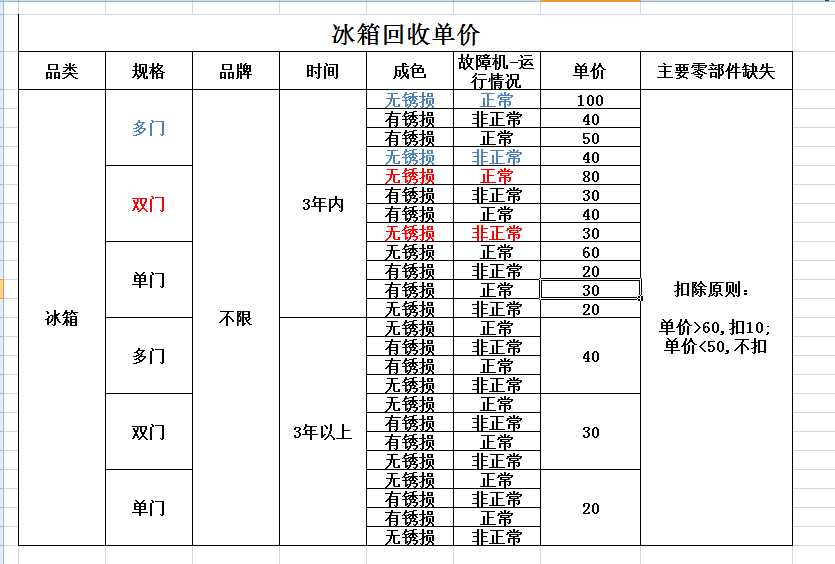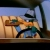php N组数据之间的组合，不能有重复的，实例数据和效果如下，PHP程序如何排列出来？

【推荐阅读】滴滴出行的数据架构和信息流处理思路解析->>>0PHP代码：

\$arr = array(
'a'=>array('a1','a2','a3'),
'b'=>array('b1','b2','b3'),
'c'=>array('c1','c2','c3'),

//..........多行......
);

fun(\$arr);
//print_r(\$res);

echo '<table width="150px" border="1">';
\$j=0;
echo "<tr>";
echo "<td>序号</td>";
foreach(\$arr as \$k=>\$v)
{
echo "<td>".\$k."</td>";
}
echo "</tr>";
foreach(\$res as \$v)
{
echo "<tr>";
\$i=0;
\$j++;
echo "<td>".\$j."</td>";
foreach(\$arr as \$value)
{
echo "<td>".\$v[\$i]."</td>";
\$i++;
}
echo "</tr>";
}
echo '</table>';

function fun(\$arr, \$tmp = array())
{

foreach(array_shift(\$arr) AS \$v)
{
\$tmp[]  = \$v;
if(\$arr)
{
fun(\$arr, \$tmp);
}
else
{
\$GLOBALS["res"][]   = \$tmp;
}
array_pop(\$tmp);
}
}